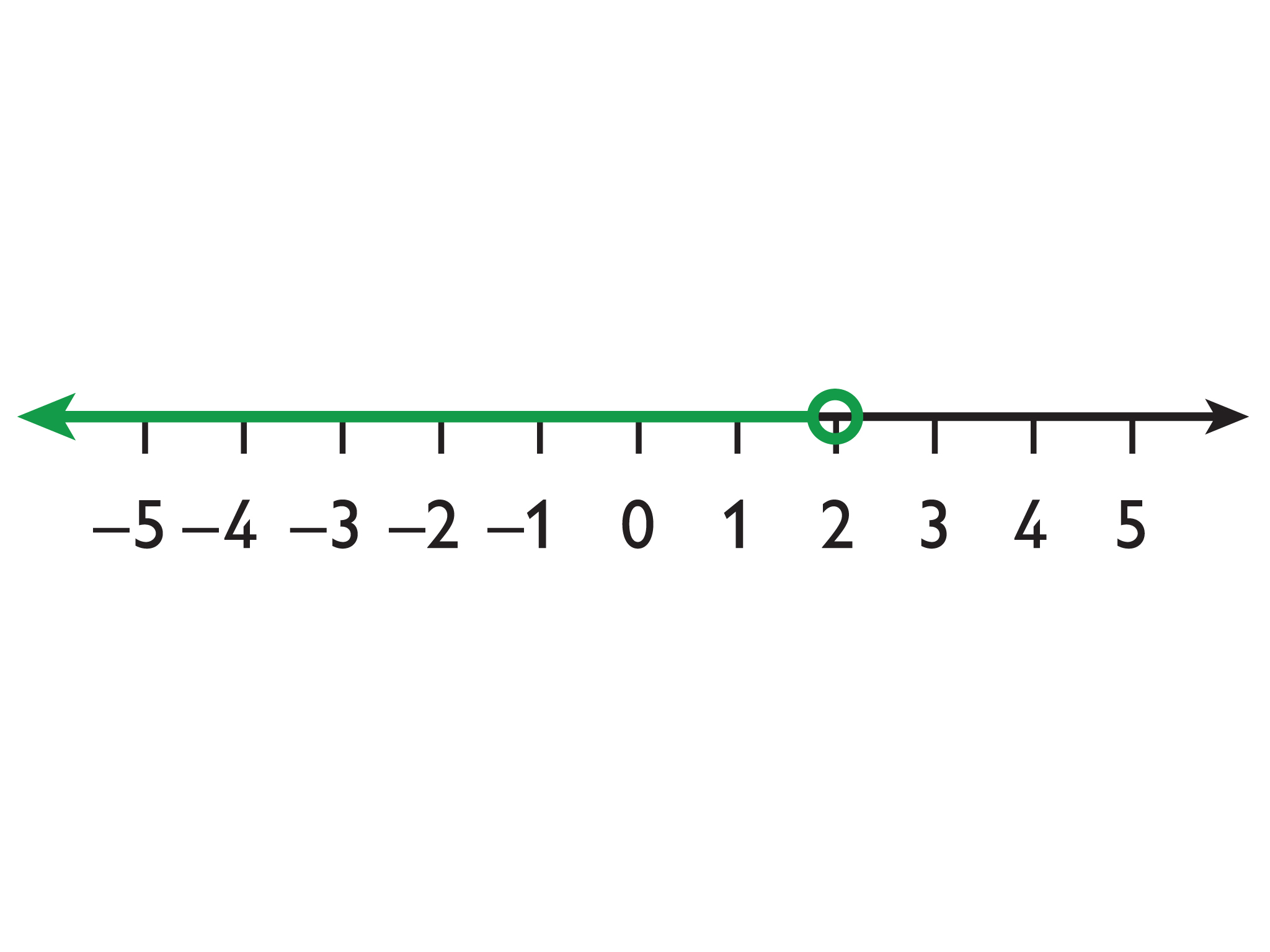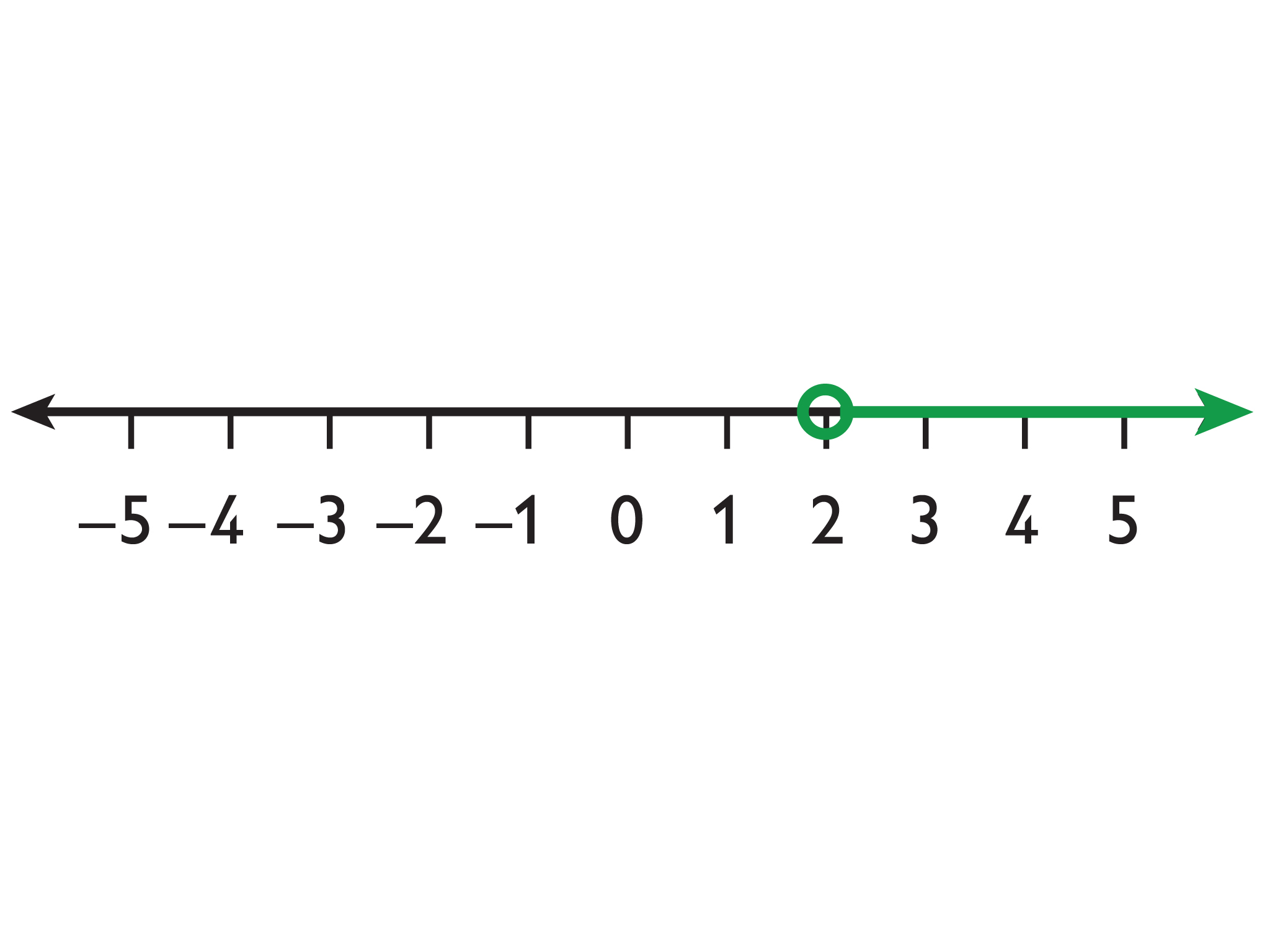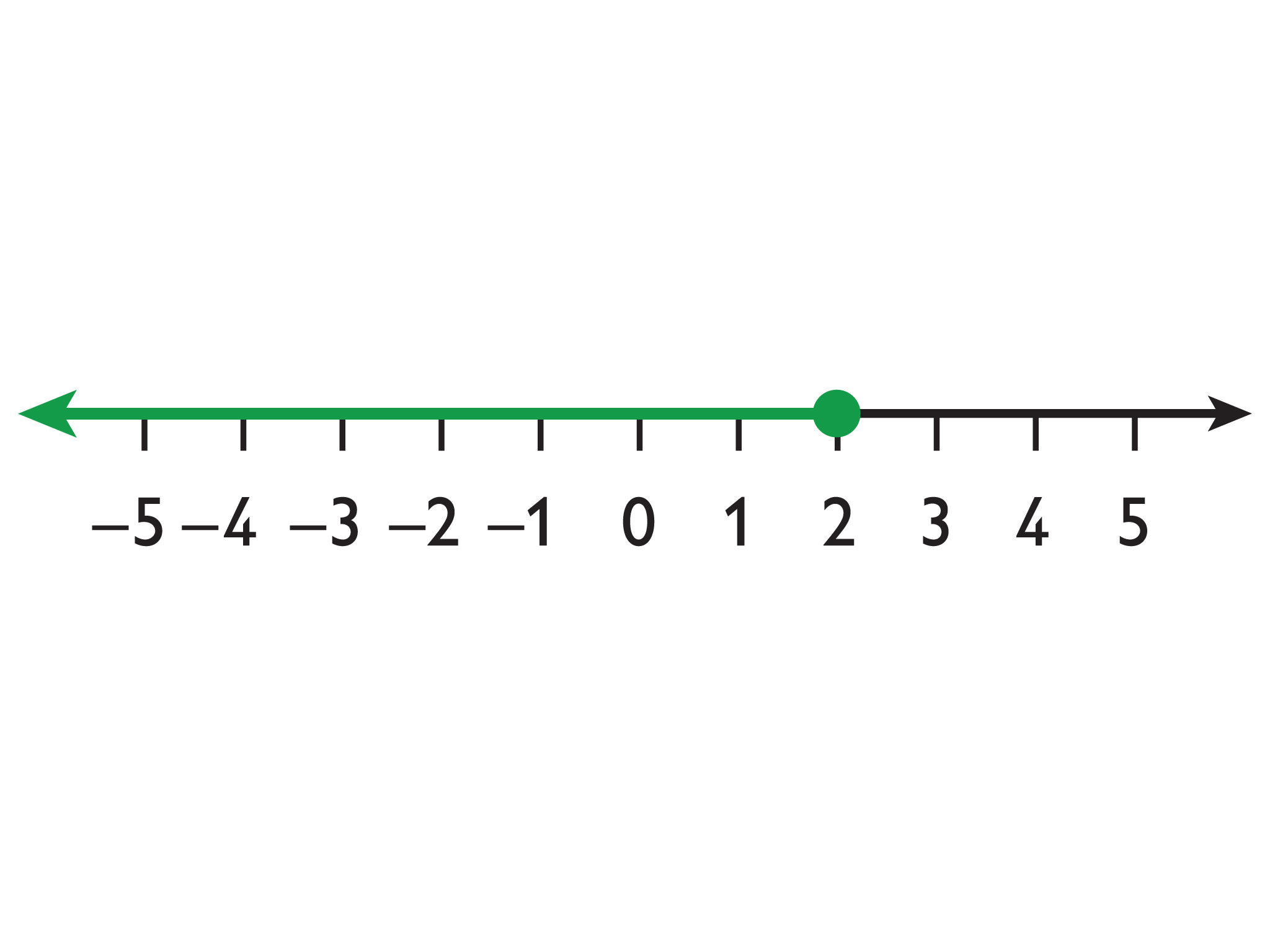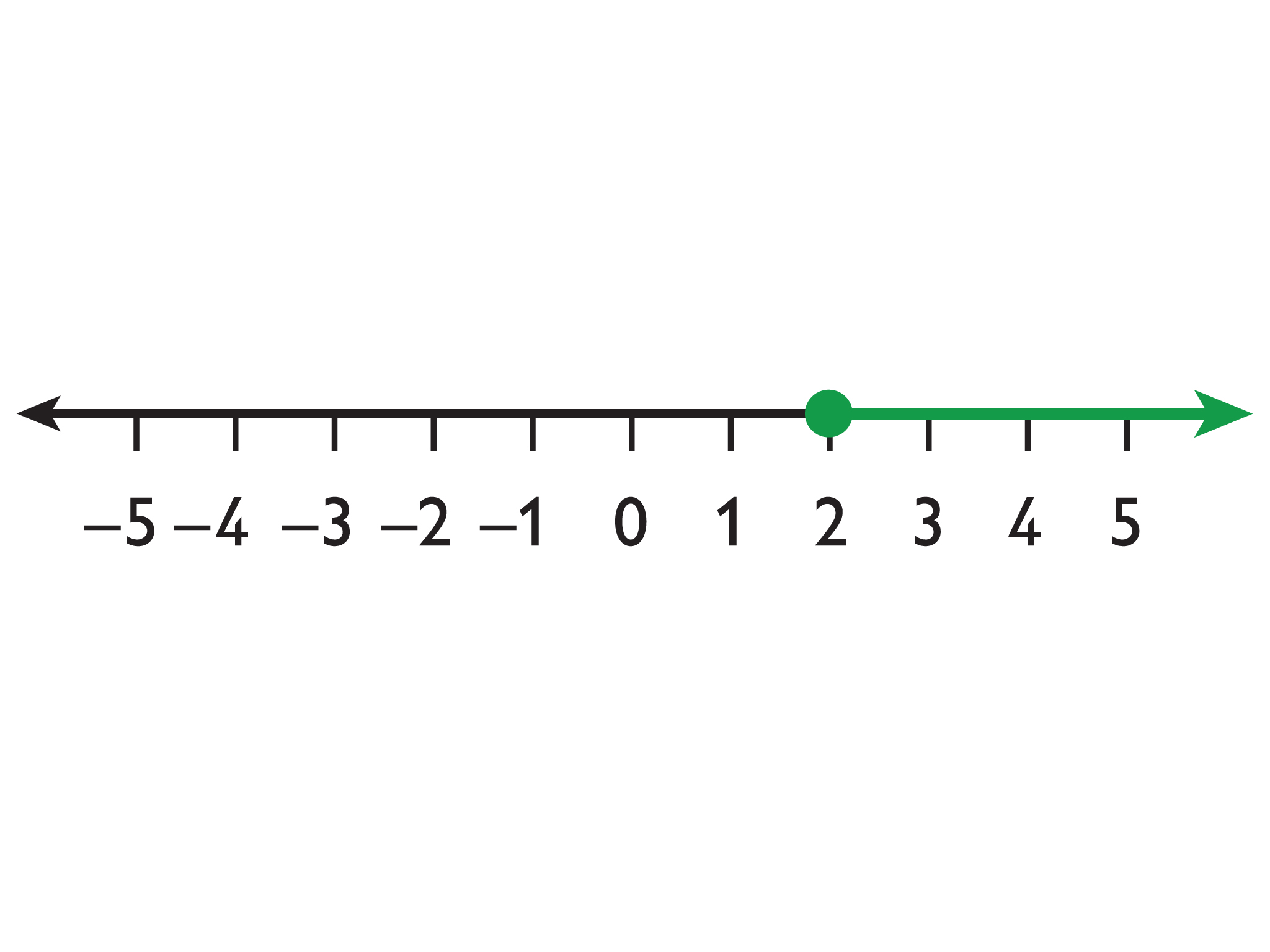# Representing An Inequality On A Number Line

Student View (Opens in new window)# Lesson Overview

Students represent inequalities on a number line, find at least one value that makes the inequality true, and write the inequality using words.

SWD:When calling on students, be sure to call on ELLs and to encourage them to actively participate. Understand that their pace might be slower or they might be shy or more reluctant to volunteer due to their weaker command of the language.

SWD:Thinking aloud is one strategy for making learning visible. When teachers think aloud, they are externalizing their internal thought processes. Doing so may provide students with insights into mathematical thinking and ways of tackling problems. It also helps to model accurate mathematical language.

# Key Concepts

Inequalities, like equations, have solutions. An arrow on the number line—pointing to the right for greater values and to the left for lesser values—can be used to show that there are infinitely many solutions to an inequality.

The solutions to x < a are represented on the number line by an arrow pointing to the left from an open circle at a.

Example: x < 2The solutions to x > a are represented on the number line with an arrow pointing to the right from an open circle at a.

Example: x > 2The solutions to xa are represented on the number line with an arrow pointing to the left from a closed circle at a.

Example: x ≤ 2The solutions to xa are represented on the number line with an arrow pointing to the right from a closed circle at a.

Example: x ≥ 2# Goals and Learning Objectives

• Represent an inequality on a number line and using words.
• Understand that inequalities have infinitely many solutions.